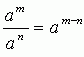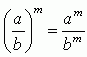Email us to get an instant 20% discount on highly effective K-12 Math & English kwizNET Programs!

#### Online Quiz (WorksheetABCD)

Questions Per Quiz = 2 4 6 8 10

### High School Mathematics - 21.16 Properties of Exponents

 Laws of exponents: am x an = am+n (am)n = amn (ab)m = am x bma0 = 1 a1 = a a-n = 1/an Direction: Solve the following problems. Also write the laws of exponents on a piece of paper.
 Q 1: Simplify, [(x)7]2 = _____?x14x7 Question 2: This question is available to subscribers only!